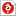검색어 입력폼

성대 열유체 연료분석 Fuel analysis,열분해 보고서

저작시기 2012.05 |등록일 2012.05.15한컴오피스 (hwp) | 4페이지 | 가격 1,000원

* 본 문서는 한글 2005 이상 버전에서 작성된 문서입니다. 한글 2002 이하 프로그램에서는 열어볼 수 없으니, 한글 뷰어프로그램(한글 2005 이상)을 설치하신 후 확인해주시기 바랍니다.

소개글

성균관대학교 열유체공학실험 연료 분석 Fuel analysis 보고서 입니다.

목차

4. Experimental result
1) Plot the mass loss curve. (%mass vs temperature)
2) Calculate the mass loss rate. (%mass)
3) Determine the onset of pyrolysis.
4) Determine the peak of pyrolysis rate.
5) Describe the phenomena taking place in each stage of pyrolysis

5. Discussion

6. Reference

본문내용

5) Describe the phenomena taking place in each stage of pyrolysis

First process ① to ② is moisture evaporating so it occur highest point of derivative curve in about 100℃. When the temperature reaches to 100℃, all of the moisture change liquid to vapor. So, we can know that this substance has 5.997 % of water component by the graph.
Second process ② to ③, when temperature increases 232.7℃ to 432.3℃, one of components that occupies largest mass percentage about 65.037% is burned out. It is volatile matter. We can see smoke when we burn the fuels. It is volatile matter combustion.

참고 자료

The experiment manual
http://en.wikipedia.org/wiki/Char